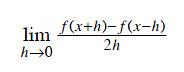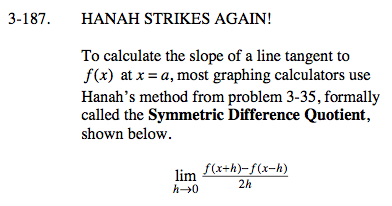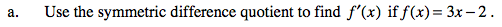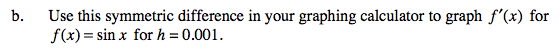### Home > CALC > Chapter 3 > Lesson 3.4.4 > Problem3-187

3-187.
1. HANAH STRIKES AGAIN!
To calculate the slope of a line tangent to f(x) at x = a, most graphing calculators use Hanah's method from problem 3-35, formally called the Symmetric Difference Quotient, shown below. Homework Help ✎1. Use the symmetric difference quotient to find f ′(x) if f(x) = 3x − 2 .

2. Use this symmetric difference in your graphing calculator to graph f ′(x) for f(x) = sin x for h = 0.001.$f'(x)=\lim_{h\rightarrow 0}\frac{(3(x+h)-2)-(3(x-h)-2)}{2h}$

Expand the numerator.
Combine like terms.
Factor out an h.
Cancel out the h.
Evaluate the limit h→0.... this is f '(x).$f'(x)\approx \lim_{h\rightarrow 0.001}=\frac{(3(x+0.001)-2)-(3(x-0.001)-2)}{2(0.001)}=\underline{ \ \ \ \ \ \ \ \ \ \ \ }$

Graph that on your calculator. Also sketch the derivative function you found in part (a). The sketches should look a lot alike.